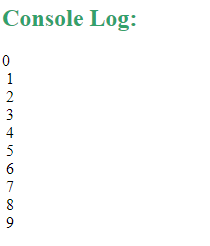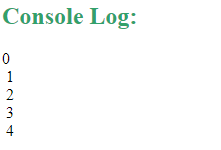# How to add a delay in a JavaScript loop?

• Last Updated : 27 Sep, 2019

JavaScript doesn’t offer any wait command to add a delay to the loops but we can do so using setTimeout method. This method executes a function, after waiting a specified number of milliseconds. Below given example illustrates how to add a delay to various loops:

• For loop:

 `for` `(let i=0; i<10; i++) { ` `   ``task(i); ` `} ` ` `  `function` `task(i) { ` `  ``setTimeout(``function``() { ` `      ``// Add tasks to do ` `  ``}, 2000 * i); ` `} `

In the code given above you have to do 2000 * i at line 8 because setTimeout method inside the loop doesn’t makes the loop pause but actually adds a delay to each iteration. Remember that all the iteration start their time together. Thus if we only do 2000 there, that will make all the iterations execute together and it will just give 2000 ms delay in the first iteration and all other iteration will happen instantly after it. Thus to avoid that we add 0 to first, 2000 to second, 4000 to third and it goes on.

Example: Below given program will print 0 to 9 in console after 2 seconds delay to each number using for loop.

 ` `

Output:• While loop: Same concept is applied to make below given while loop.

 `let i = 0; ` `while` `(i < 10) { ` `  ``task(i); ` `   ``i++; ` `} ` `function` `task(i) { ` `  ``setTimeout(``function``() { ` `      ``// Add tasks to do ` `  ``}, 2000 * i); ` `} `

Example: Below given program will print 0 to 9 in console after 2 seconds delay to each number using while loop.

 ` `

Output:• Do-while loop: Same concept is applied to make below given do-while loop.

 `let i = 0; ` `do` `{ ` `  ``task(i); ` `  ``i++; ` `} ``while` `(i < 5); ` `function` `task(i) { ` `  ``setTimeout(``function``() { ` `    ``// Add tasks to do ` `  ``}, 2000 * i); ` `} `

Example: Below given program will print 0 to 9 in console after 2 seconds delay to each number using do-while loop.

 ` `

Output:My Personal Notes arrow_drop_up
Recommended Articles
Page :